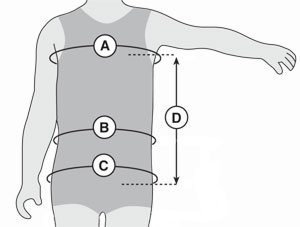# Custom Sizing

For custom fitting, take measurements as indicated (Measurements to the nearest 1/8" are most helpful). When ordering, note these exact measurements.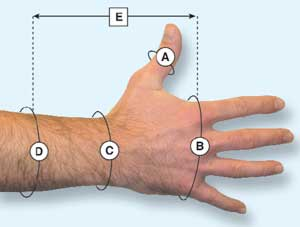#### Wrist

1. A. Circumference at thumb IP.
2. B. Circumference at MCPs.
3. C. Circumference at wrist.
4. D. Circumference at proximal end.
5. E. Desired length from index MCP to proximal end.

Specify right or left hand.

See our Hand Splint Sizing Guide for additional measuring details, including
for a Volar Pan Extension.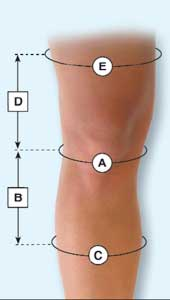#### Knee

1. A. Measure circumference at mid patella.
2. B. Using anterior side, measure mid patella to desired distal end.
3. C. Measure circumference at distal end.
4. D. Using anterior side, measure mid patella to desired proximal end.
5. E. Measure circumference at proximal end.
For measurement (E), tilt tape down about 1.5" at posterior to
reflect actual proximal line of sleeve.

NOTE: Additional incremental circumference measurements may be needed for very long knee supports.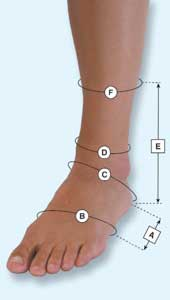#### Ankle

1. A. Length from heel to just proximal of MTPs.
2. B. Circumference at desired distal end of splint.
3. C. Circumference at instep.
4. D. Circumference at ankle.
5. E. Length from floor to desired proximal end of splint.
6. F. Circumference at proximal end of splint.

Provide patient shoe size for custom ankle products.

Specify right or left foot.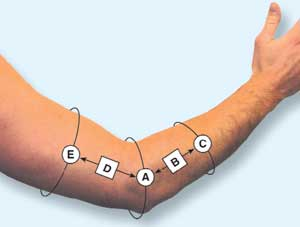#### Elbow

1. A. Circumference at elbow.
2. B. Using lateral side, desired distal length from elbow.
3. C. Circumference at distal end.
4. D. Using lateral side, desired proximal length from elbow.
5. E. Circumference at proximal end.

Specify right or left arm.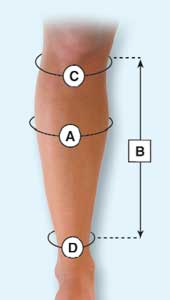#### Calf

1. A. Measure circumference at widest part of calf.
2. B. State desired overall length of support.

3. Once length of support is determined ...

4. C. Measure circumference of calf at top of support.
5. D. Measure circumference of calf at bottom of support.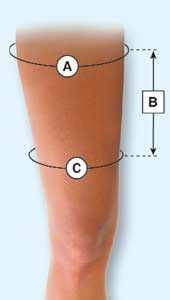#### Groin/Thigh

1. A. Measure circumference of thigh at widest points.
2. B. Using medial side, state desired overall length of support.

3. Once length of support is determined ...

4. C. Measure circumference of thigh at bottom of support.

Specify right or left thigh.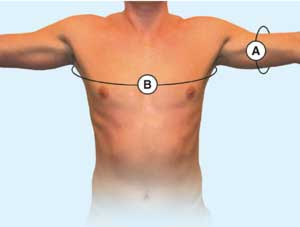#### Shoulder

1. A. Measure circumference at bicep.
2. B. Measure circumference around chest.

Specify right or left shoulder.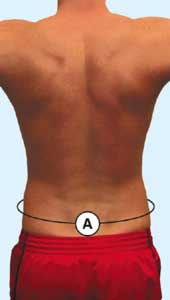#### Lumbar

1. A. Measure circumference at lumbar area.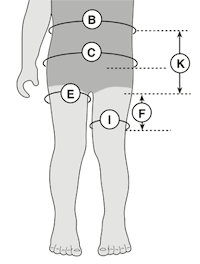#### Neoprene Shorts

1. B. Measure circumference at waist.
2. C. Measure circumference at hips.
3. E. Measure circumference at thigh.
4. F. Measure inseam length from crotch to desired distal end of shorts.
5. I. Measure circumference of thigh at desired distal end.
6. K. Measure length from crotch to top of shorts.